# MSBSHSE Solutions For Class 8 Maths Part 1 Chapter 9- Discount and Commission

MSBSHSE Solutions For Class 8 Maths Part 1 Chapter 9 – Discount and Commission are available here for students preparing easily and score good marks in their board examinations. To achieve a good academic score in Mathematics, students are required to practise all the questions of each and every exercise present in this chapter. Here, in this chapter, we shall learn concepts related to discount, commission and rebate. The solutions are designed by our subject experts, to help understand the concepts and methods to solve problems in a shorter period and also to boost the confidence levels among students. MSBSHSE Solutions for Class 8 are of immense help to students aiming to secure a good academic score in the board exams. Maharashtra State Board Class 8 Textbooks Part 1 mainly focuses on learning various Mathematical tricks and shortcuts for quick and easy calculations. Students can easily get the pdf of Maharashtra Board Solutions for Class 8 Maths Chapter 9 Discount and Commission, from the links mentioned.

## Download the PDF of Maharashtra Board Solutions For Class 8 Maths Part 1 Chapter 9- Discount and Commission.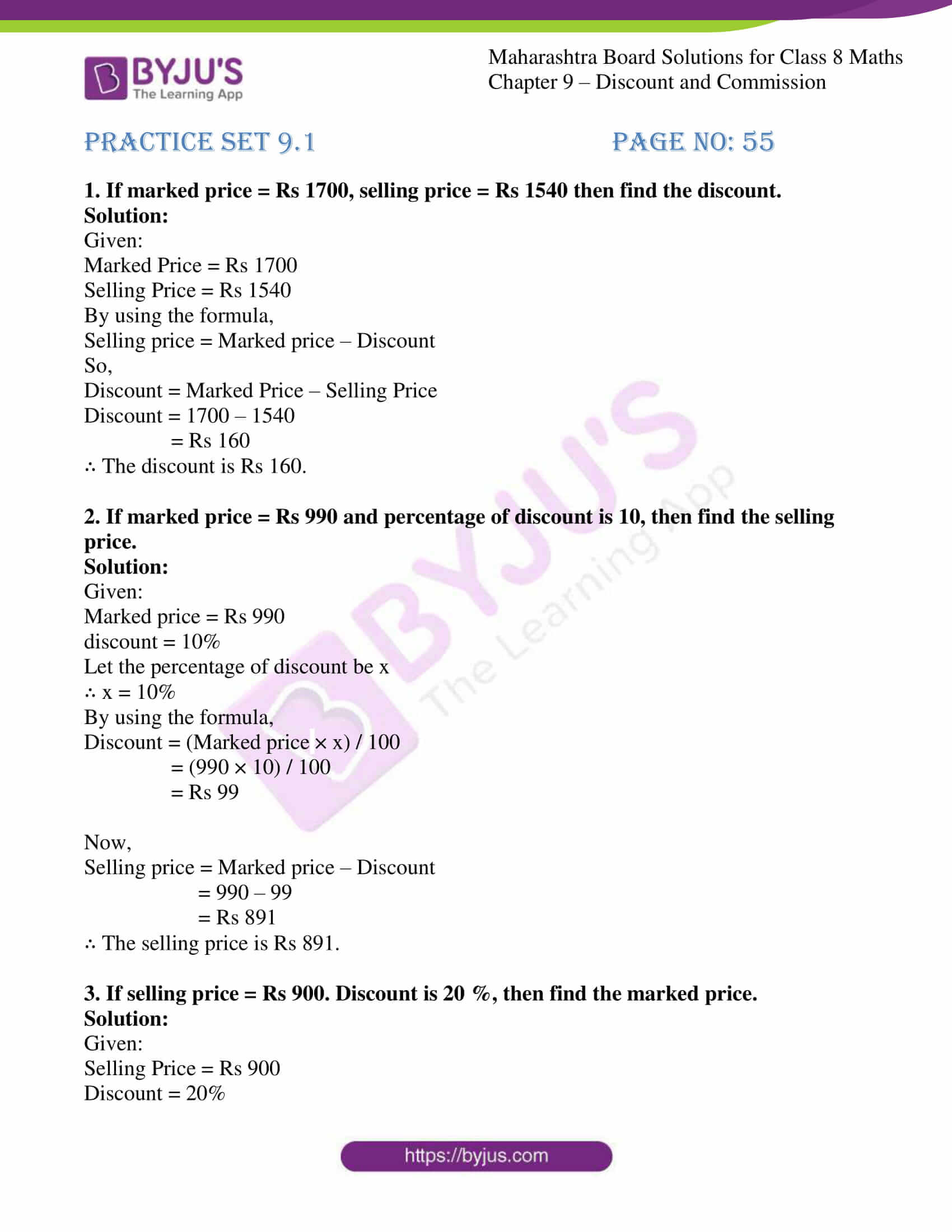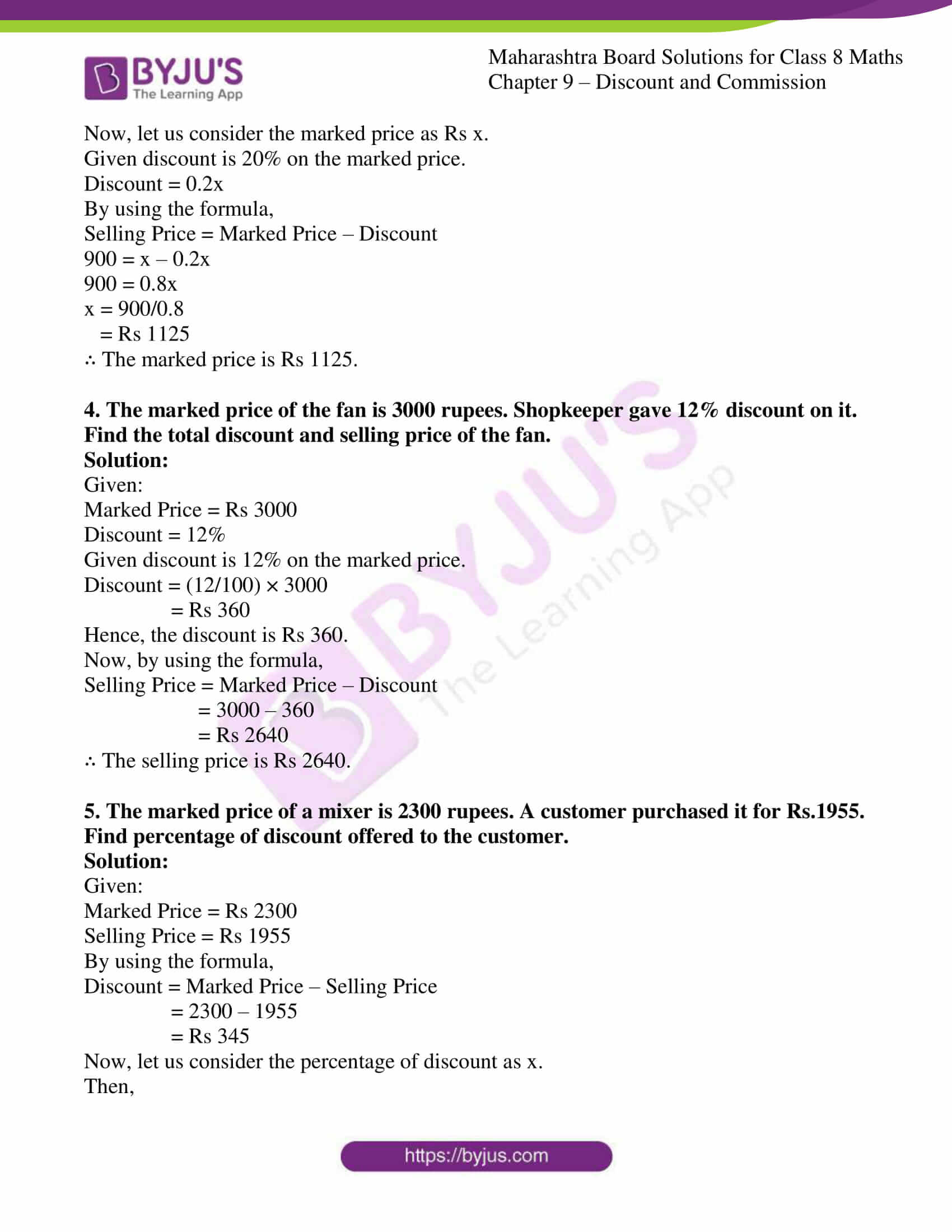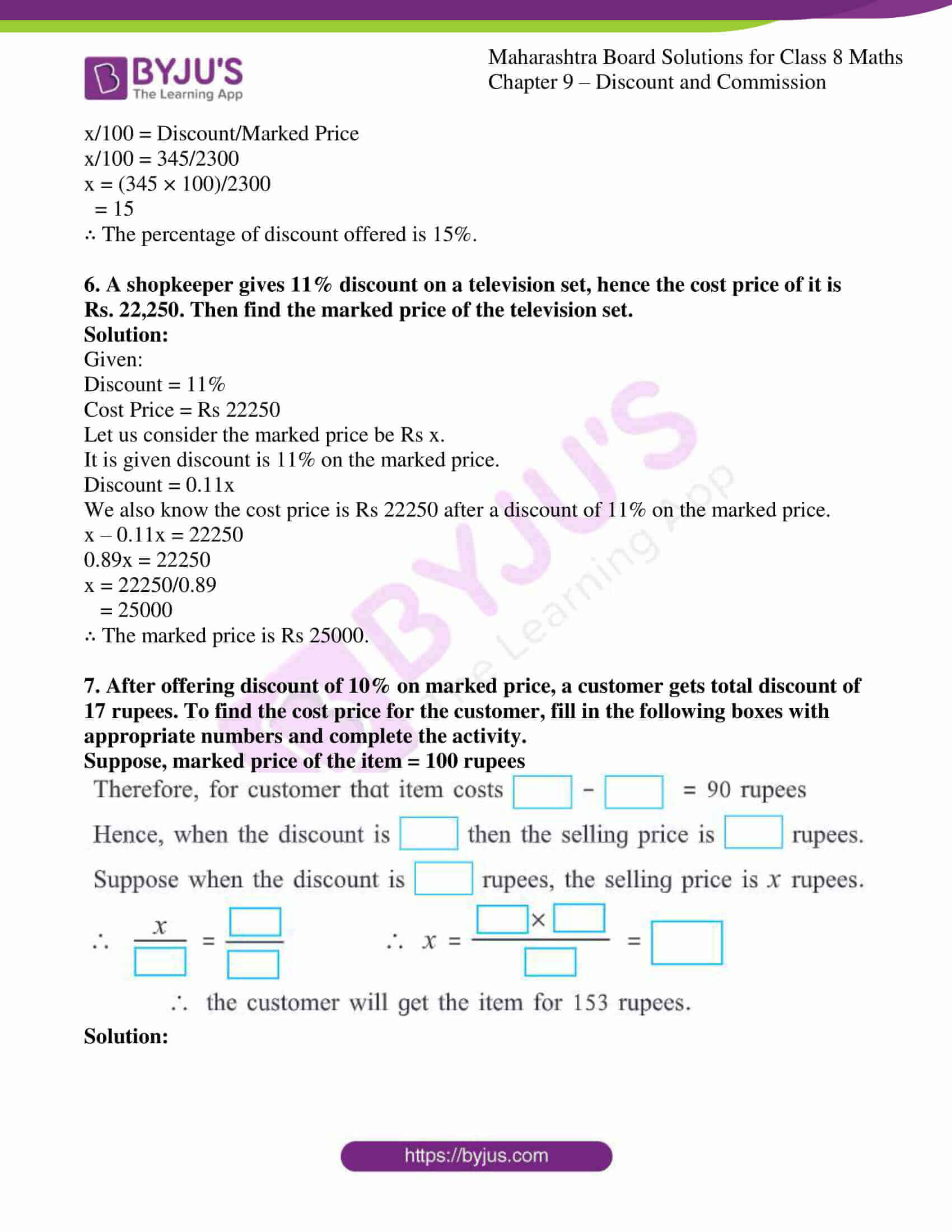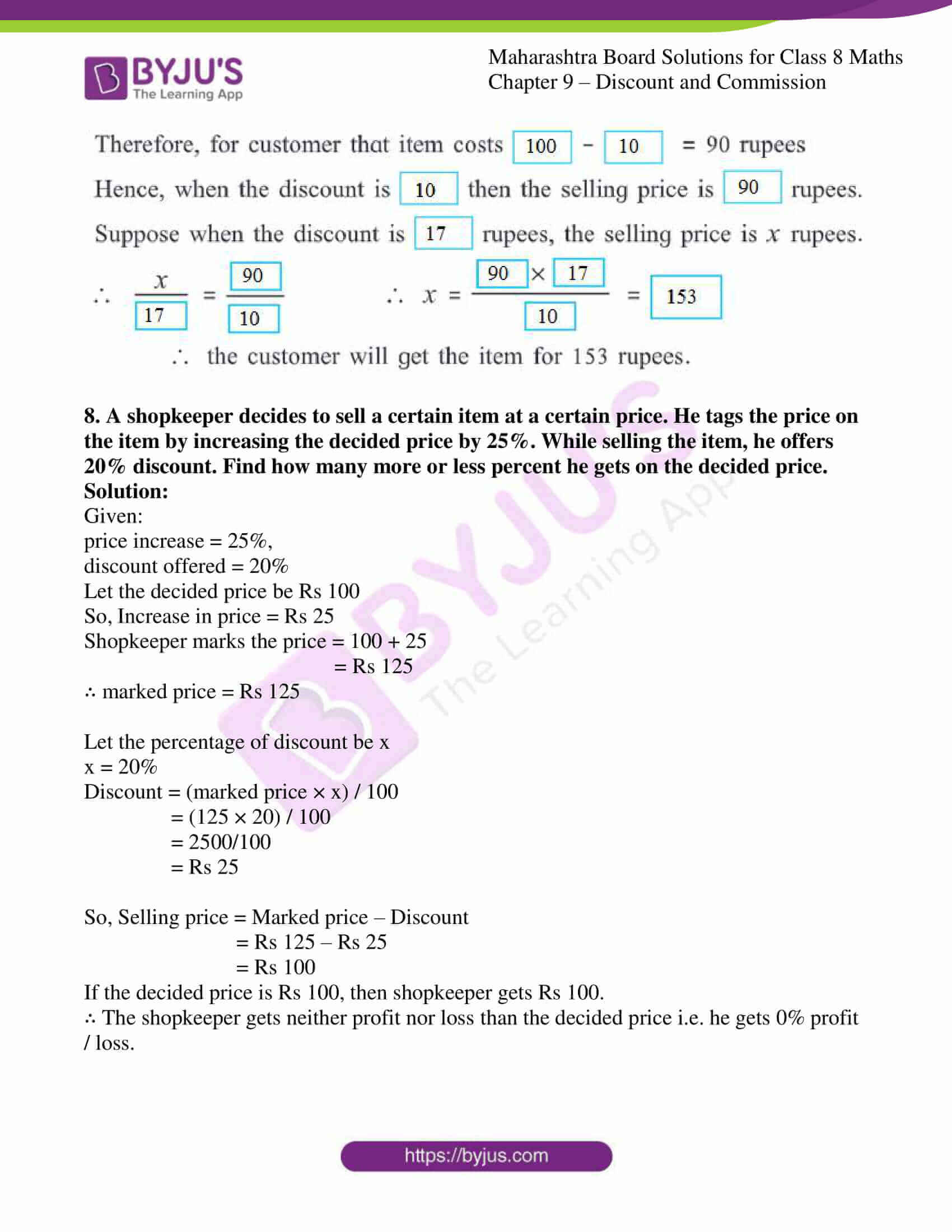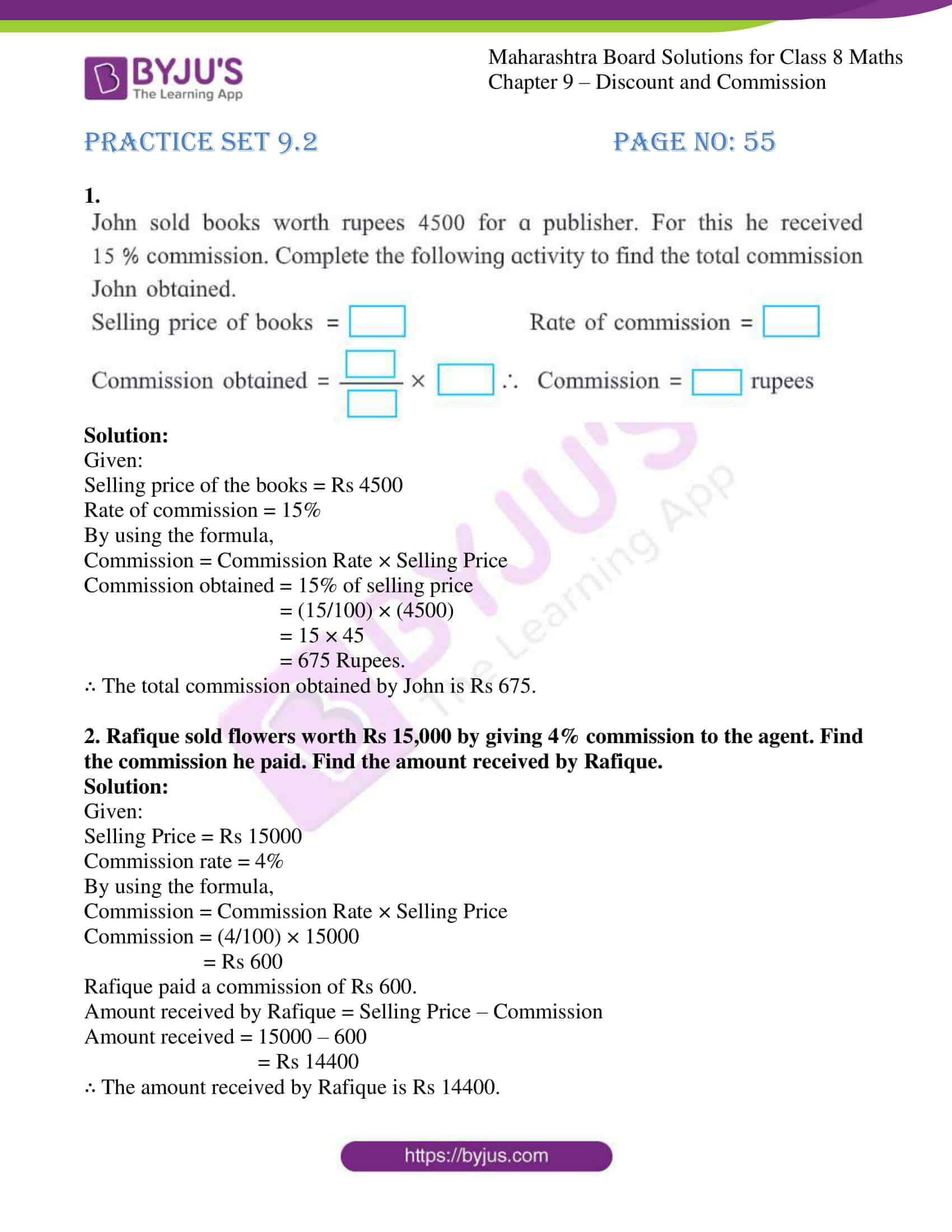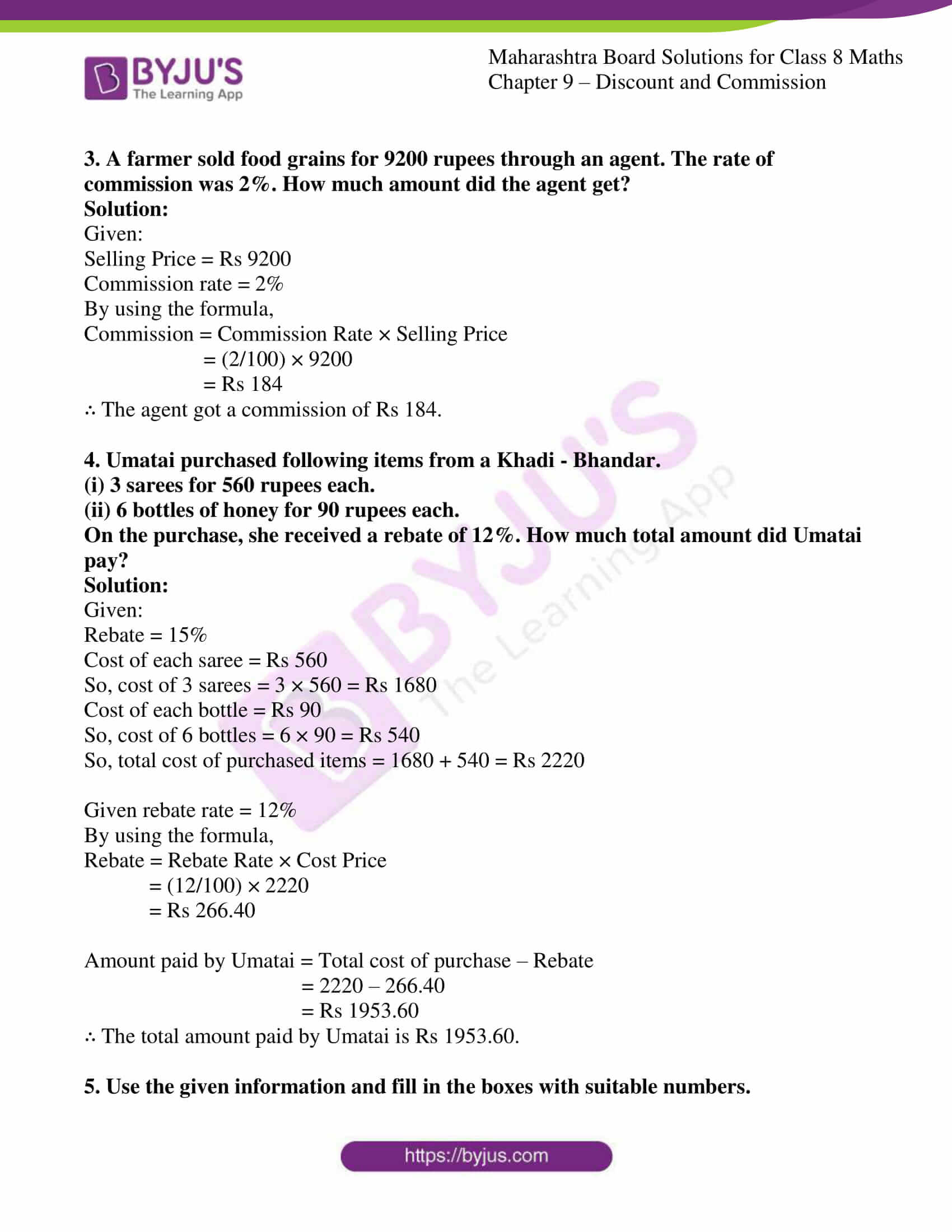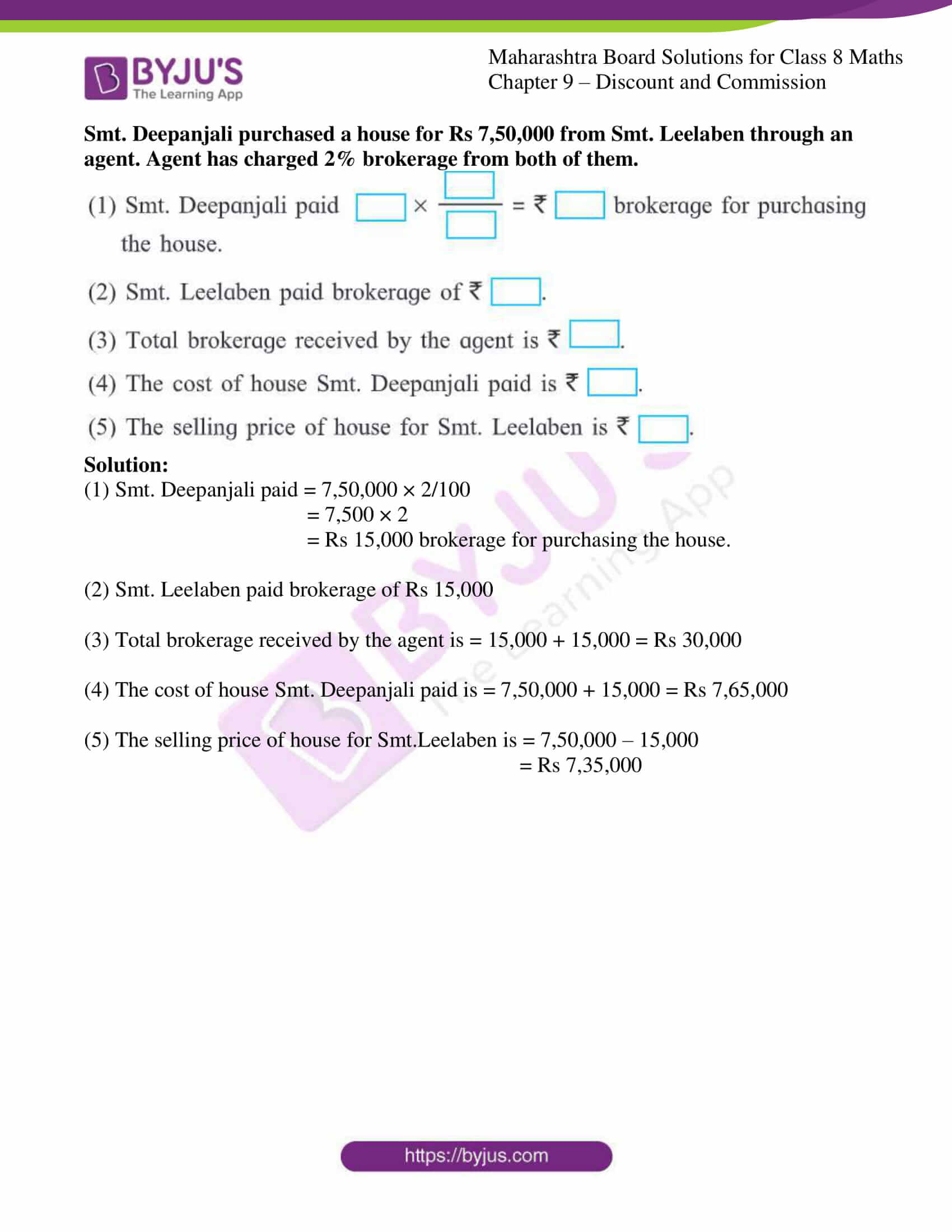### Access answers to Maharashtra Board Solutions For Class 8 Maths Part 1 Chapter 9- Discount and Commission.

Practice set 9.1 PAGE NO: 55

1. If marked price = Rs 1700, selling price = Rs 1540 then find the discount.

Solution:

Given:

Marked Price = Rs 1700

Selling Price = Rs 1540

By using the formula,

Selling price = Marked price – Discount

So,

Discount = Marked Price – Selling Price

Discount = 1700 – 1540

= Rs 160

∴ The discount is Rs 160.

2. If marked price = Rs 990 and percentage of discount is 10, then find the selling price.

Solution:

Given:

Marked price = Rs 990

discount = 10%

Let the percentage of discount be x

∴ x = 10%

By using the formula,

Discount = (Marked price × x) / 100

= (990 × 10) / 100

= Rs 99

Now,

Selling price = Marked price – Discount

= 990 – 99

= Rs 891

∴ The selling price is Rs 891.

3. If selling price = Rs 900. Discount is 20 %, then find the marked price.

Solution:

Given:

Selling Price = Rs 900

Discount = 20%

Now, let us consider the marked price as Rs x.

Given discount is 20% on the marked price.

Discount = 0.2x

By using the formula,

Selling Price = Marked Price – Discount

900 = x – 0.2x

900 = 0.8x

x = 900/0.8

= Rs 1125

∴ The marked price is Rs 1125.

4. The marked price of the fan is 3000 rupees. Shopkeeper gave 12% discount on it. Find the total discount and selling price of the fan.

Solution:

Given:

Marked Price = Rs 3000

Discount = 12%

Given discount is 12% on the marked price.

Discount = (12/100) × 3000

= Rs 360

Hence, the discount is Rs 360.

Now, by using the formula,

Selling Price = Marked Price – Discount

= 3000 – 360

= Rs 2640

∴ The selling price is Rs 2640.

5. The marked price of a mixer is 2300 rupees. A customer purchased it for Rs.1955. Find percentage of discount offered to the customer.

Solution:

Given:

Marked Price = Rs 2300

Selling Price = Rs 1955

By using the formula,

Discount = Marked Price – Selling Price

= 2300 – 1955

= Rs 345

Now, let us consider the percentage of discount as x.

Then,

x/100 = Discount/Marked Price

x/100 = 345/2300

x = (345 × 100)/2300

= 15

∴ The percentage of discount offered is 15%.

6. A shopkeeper gives 11% discount on a television set, hence the cost price of it is Rs. 22,250. Then find the marked price of the television set.

Solution:

Given:

Discount = 11%

Cost Price = Rs 22250

Let us consider the marked price be Rs x.

It is given discount is 11% on the marked price.

Discount = 0.11x

We also know the cost price is Rs 22250 after a discount of 11% on the marked price.

x – 0.11x = 22250

0.89x = 22250

x = 22250/0.89

= 25000

∴ The marked price is Rs 25000.

7. After offering discount of 10% on marked price, a customer gets total discount of 17 rupees. To find the cost price for the customer, fill in the following boxes with appropriate numbers and complete the activity.

Suppose, marked price of the item = 100 rupees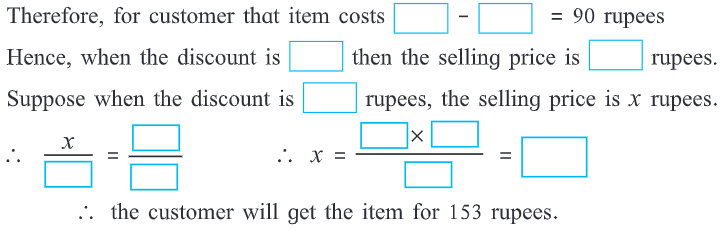Solution: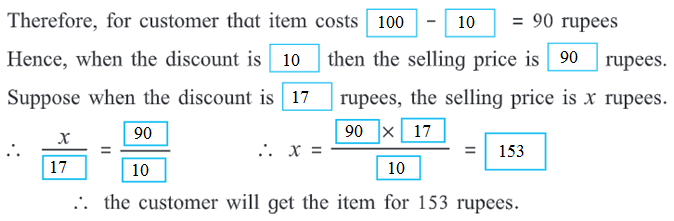8. A shopkeeper decides to sell a certain item at a certain price. He tags the price on the item by increasing the decided price by 25%. While selling the item, he offers 20% discount. Find how many more or less percent he gets on the decided price.

Solution:

Given:

price increase = 25%,

discount offered = 20%

Let the decided price be Rs 100

So, Increase in price = Rs 25

Shopkeeper marks the price = 100 + 25

= Rs 125

∴ marked price = Rs 125

Let the percentage of discount be x

x = 20%

Discount = (marked price × x) / 100

= (125 × 20) / 100

= 2500/100

= Rs 25

So, Selling price = Marked price – Discount

= Rs 125 – Rs 25

= Rs 100

If the decided price is Rs 100, then shopkeeper gets Rs 100.

∴ The shopkeeper gets neither profit nor loss than the decided price i.e. he gets 0% profit / loss.

Practice set 9.2 PAGE NO: 55

1.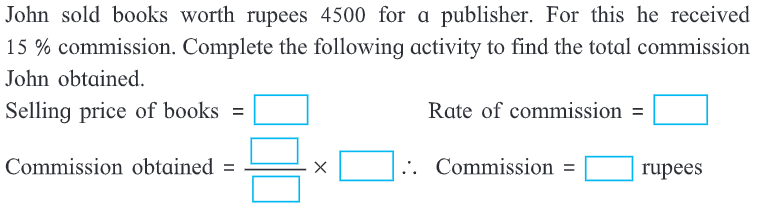Solution:

Given:

Selling price of the books = Rs 4500

Rate of commission = 15%

By using the formula,

Commission = Commission Rate × Selling Price

Commission obtained = 15% of selling price

= (15/100) × (4500)

= 15 × 45

= 675 Rupees.

∴ The total commission obtained by John is Rs 675.

2. Rafique sold flowers worth Rs 15,000 by giving 4% commission to the agent. Find the commission he paid. Find the amount received by Rafique.

Solution:

Given:

Selling Price = Rs 15000

Commission rate = 4%

By using the formula,

Commission = Commission Rate × Selling Price

Commission = (4/100) × 15000

= Rs 600

Rafique paid a commission of Rs 600.

Amount received by Rafique = Selling Price – Commission

Amount received = 15000 – 600

= Rs 14400

∴ The amount received by Rafique is Rs 14400.

3. A farmer sold food grains for 9200 rupees through an agent. The rate of commission was 2%. How much amount did the agent get?

Solution:

Given:

Selling Price = Rs 9200

Commission rate = 2%

By using the formula,

Commission = Commission Rate × Selling Price

= (2/100) × 9200

= Rs 184

∴ The agent got a commission of Rs 184.

4. Umatai purchased following items from a Khadi – Bhandar.
(i) 3 sarees for 560 rupees each.
(ii) 6 bottles of honey for 90 rupees each.
On the purchase, she received a rebate of 12%. How much total amount did Umatai pay?

Solution:

Given:

Rebate = 15%

Cost of each saree = Rs 560

So, cost of 3 sarees = 3 × 560 = Rs 1680

Cost of each bottle = Rs 90

So, cost of 6 bottles = 6 × 90 = Rs 540

So, total cost of purchased items = 1680 + 540 = Rs 2220

Given rebate rate = 12%

By using the formula,

Rebate = Rebate Rate × Cost Price

= (12/100) × 2220

= Rs 266.40

Amount paid by Umatai = Total cost of purchase – Rebate

= 2220 – 266.40

= Rs 1953.60

∴ The total amount paid by Umatai is Rs 1953.60.

5. Use the given information and fill in the boxes with suitable numbers.
Smt. Deepanjali purchased a house for Rs 7,50,000 from Smt. Leelaben through an agent. Agent has charged 2% brokerage from both of them.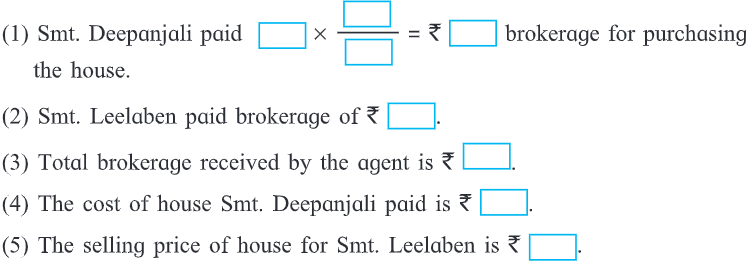Solution:

(1) Smt. Deepanjali paid = 7,50,000 × 2/100

= 7,500 × 2

= Rs 15,000 brokerage for purchasing the house.

(2) Smt. Leelaben paid brokerage of Rs 15,000

(3) Total brokerage received by the agent is = 15,000 + 15,000 = Rs 30,000

(4) The cost of house Smt. Deepanjali paid is = 7,50,000 + 15,000 = Rs 7,65,000

(5) The selling price of house for Smt.Leelaben is = 7,50,000 – 15,000

= Rs 7,35,000

Highly experienced subject experts at BYJU’S having vast knowledge of concepts have developed the solutions, which match the understanding ability of the students. Chapter 9 discusses Discount and Commission, where students can refer to MSBSHSE Solutions to score full marks in the examination. Many such exercise problems are given for students to practise and excel in their board examination.

## Frequently Asked Questions on Maharashtra State Board Solutions for Class 8 Maths Chapter 9 Discount and Commission

### Are these Maharashtra State Board Class 8 Maths Chapter 9 Solutions important?

Yes, these Maharashtra State Board Solutions for Class 8 Maths Chapter 9 Discount and Commission  are very important. These solutions are devised in such a way that  designed in a way that every student can easily grasp the concept into their minds and clarify whatever doubts they have about the topic. The solutions provided are self-explanatory, and the step-by-step guidance will help the students. Also, the board exam aspirants can assess their learning abilities and exam preparations with these solutions.